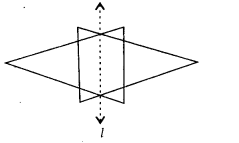# MP Board Class 6th Maths Solutions Chapter 13 Symmetry Ex 13.1

## MP Board Class 6th Maths Solutions Chapter 13 Symmetry Ex 13.1

Question 1.
List any four symmetrical objects from your home or school.
Solution:
Notebook. Blackboard, Glass, Inkpot.

Question 2.
For the given figure, which one is the mirror line, l1, or l2?Solution:
l2 is the mirror line as both sides of the line are symmetric.Question 3.
Identify the shapes given below. Check whether they are symmetric or not. Draw the line of symmetry as well.Solution:
(a) Symmetric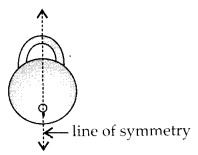(b) Symmetric(c) Not Symmetric
(d) Symmetric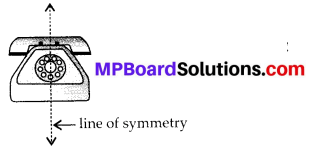(e) Symmetric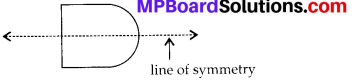(f) SymmetricQuestion 4.
Copy the following on a squared paper. A square paper is what you would have used in your arithmetic notebook in earlier classes. Then complete them such that the dotted line is the line of symmetry.Solution: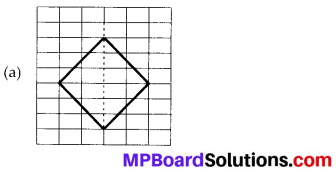Question 5.
In thefigure, l is the line of symmetry. Complete the diagram to make it symmetric.Solution: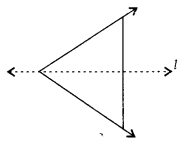Question 6.
In the figure, l is the line of symmetry. Draw the image of the triangle and complete the diagram so that it becomes symmetric.Solution: